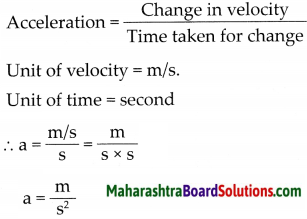Balbharti Maharashtra State Board Class 7 Science Solutions Chapter 7 Motion, Force and Work Notes, Textbook Exercise Important Questions and Answers.

## Maharashtra State Board Class 7 Science Solutions Chapter 7 Motion, Force and Work

Class 7 Science Chapter 7 Motion, Force and Work Textbook Questions and Answers

1. Fill ¡n the blanks with the proper words from the brackets.
(stationary, zero, changing, constant, displacement, velocity, speed. acceleration, stationary but not zero. inc reuses)

Question a.
If a body traverses a distance in direct proportion to the time, the speed of the body is ……………… .
constant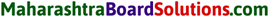Question b.
If a body is moving with a constant velocity, its acceleration is ……………… .
zero

Question c.
……………. is a scalar quantity.
Speed

Question d.
…………….. is the distance traversed by a body in a particular direction in unit time.
Velocity

2. Observe the figure and answer the questions.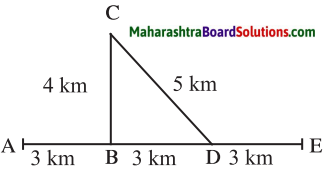Sachin and Sarneer started on a motorbike from place A, took the turn at 13, did a task at C, travelled by the route CD to D and then went on to E. Altogether, they took one hour for this journey. Find out the actual distance traversed by them and the displacement from A to E. From this, deduce their speed. What was their velocity from A to E in the direction AE’? Can this velocity be called average velocity?

Question a.
Observe the figure and answer the questionsSachin and Sarneer started on a motorbike from place A, took the turn at 13, did a task at C, travelled by the route CD to D and then went on to E. Altogether, they took one hour for this journey. Find out the actual distance traversed by them and the displacement from A to E. From this, deduce their speed. What was their velocity from A to E in the direction AE’? Can this velocity be called average velocity?
1. Actual distance = $$\overline{\mathrm{AB}}$$ + $$\overline{\mathrm{BC}}$$ + $$\overline{\mathrm{CD}}$$ + $$\overline{\mathrm{DE}}$$ = 3 + 4 + 5 + 3
Actual distance = 15 km

2. Displacement = $$\overline{\mathrm{AB}}$$ + $$\overline{\mathrm{BD}}$$ + $$\overline{\mathrm{DE}}$$
= 3 + 3 + 3
Displacement = 9 km

3. Speed = $$\frac{\text { Distance travelled }}{\text { Total time }}$$
Distance = 15 km = 15 × 1000 = 15000 m
Time = 1 hr = 1 × 60 × 60 = 3600 sec.
s = $$\frac{15000}{3600}$$ or s = $$\frac{15 \mathrm{~km}}{1 \text { hour }}$$ = 15km/hour
= 4.16 m/sec. or 15 km/hour

4. Velocity = $$\frac{\text { Distance travelled }}{\text { Total time }}$$
Displacement = 9 km = 9 × 1000 = 9000 m
Time = 1 hr = 1 × 60 × 60 = 3600 sec
V = $$\frac{9000}{3600}$$ or V = $$\frac{9 \mathrm{~km}}{1 \text { hour }}$$ = 9 km/hour
= 2.5 m/sec. or 9 km/hour

5. Yes, this velocity can be called as average velocity.3. From the groups B and C, choose the proper words, for each of the words in group A.

Question a.
From the groups B and C, choose the proper words, for each of the words in group A.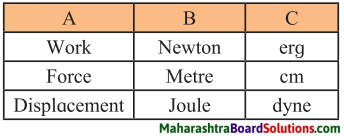Group ‘A’ Group B’ Group ‘C’ Work Joule erg Force Newton dyne Displacement Metre cm

4. A bird sitting on a wire, flies, circles around and comes back to its perch. Explain the total distance it traversed during its flight and its eventual displacement.

Question a.
The total distance the bird has traversed is the length of the distance covered by circling, but the eventual displacement are the bird is zero as its initial and final position are one and the same.5. Explain the following concepts in your own words with everyday examples: force, work, displacement, velocity, acceleration, distance.

Question a.
Explain the following concepts in your own words with everyday examples: force, work, displacement, velocity, acceleration, distance.
1. Force: The interaction that brings about the acceleration is called force.
e.g: An ox is pulling a cart, applying brakes to a bicycle, lifting heavy iron object with a crane.

2. Work: When an object is displaced by applying a force on it, work is said to be done.
e.g: A bucketful of water is to be drawn from a well and taken to the home by walking from well to home.

3. Displacement: The minimum distance
traversed by a moving body in one direction from the original point to reach the final point is called displacement.
e.g: A rolling of a ball from point A to point B in the same direction.

4. Velocity: Velocity is the distance traversed by a body in a specific direction in unit time.
e.g: A truck is covering a distance of 40km from A to D in a straight line in 1 hour.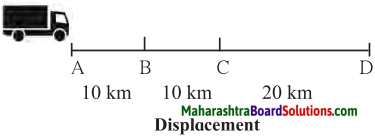5. Acceleration: It is change in velocity per second. It can be deduced.
Acceleration = $$\frac{\text { Change in velocity }}{\text { Time taken for change }}$$
e.g: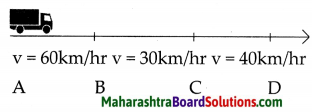(i) In the above example a truck covered the distance AB at velocity of 60 km/hr, BC at 30 km/hr and CD at 40 km/hr. (ii) It means that the velocity for the distance CD is greater than the velocity for the distance BC. (iii) From the number of seconds required for this change in velocity to take place, the change in velocity per second can be deduced. This is called acceleration (iv) Distance: The length of the route actually traversed by a moving body irrespective of the direction is called distance.
e.g: Ranjit travelled 1km. from his home to school.

6. A ball is rolling from A to D on a flat and smooth surface. Its speed is 2 cm/s. On reaching B, it was pushed continuously up to C. On reaching D from C, its speed had become 4 cm/s. It took 2 seconds for it to go from B to C. What is the acceleration of the ball as it goes from B to C.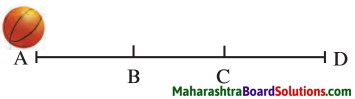Question a.
A ball is rolling from A to D on a flat and smooth surface. Its speed is 2 cm/s. On reaching B, it was pushed continuously up to C. On reaching D from C, its speed had become 4 cm/s. It took 2 seconds for it to go from B to C. What is the acceleration of the ball as it goes from B to C.As its initial and final positions are one and the same.
Initial Velocity = 2 cm/s.
Final Velocity = 4 cm/s
Time taken for the change in velocity from B to
D = 4 cm/s – 2 cm/s = 2 cm/s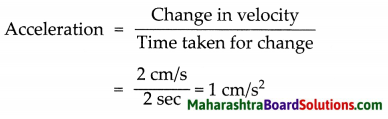7. Solve the following problems.

Question a.
A force of 1000 N was applied to stop a car that was moving with a constant velocity. The car stopped after moving through 10m. How much is the work done?
Force (F) = 1000 N
displacement (s) = 10m
work done (W) = ?
W = Fs
= 1000 × 10
W = 10,000 JouleQuestion b.
A cart with mass 20 kg went 50 m in a straight line on a plain and smooth road when a force of 2 N was applied to it. How much work was done by the force?
Force (F) = 2 N
Displacement (s) = 50 m
Work done (W) = ?
W = Fs
= 2 × 50
W = 100 Joule

Project:

Question a.
Collect information about the study made by Sir Isaac Newton regarding force and acceleration and discuss it with your teacher.

Class 7 Science Chapter 7 Motion, Force and Work Important Questions and Answers

Fill in blanks:

Question 1.
Displacement is a …………. quantity.
vector

Question 2.
The …………. of an object can change even while it is moving along a straight line.
velocityQuestion 3.
The …………. velocity can be different at different times.
instantaneous

Question 4.
Change in velocity per second is called …………. .
acceleration

Question 5.
The interaction that brings about the acceleration is called …………. .
force

Question 6.
The scientist …………. was the first to study force and the resulting acceleration.
Sir Isaac Nezvton

Question 7.
Ability to do work is called …………. .
EnergyQuestion 8.
W = …………. × S.
F

Question 9.
Unit of work is …………. and …………. .
Joule, erg

Question 10.
Unit of force is …………. and …………. .
Newton, dyne

Question 11.
Force is a …………. quantity.
vector

Question 12.
The velocity at a particular time is called …………. velocity.
instantaneousQuestion 13.
The …………. of a body is the distance traversed per unit time.
speed

Question 14.
Unit of acceleration is …………. and …………. .
m/s2 and cm/s2

Question 15.
Force is measured by the …………. that it produces.
acceleration

Question 16.
Work done by a body with no displacement will be …………. .
zero

Say whether True or False, correct the false 1 statements:

Question 1.
Velocity is distance travelled per unit of time.
False. Speed is distance travelled per unit of timeQuestion 2.
In displacement, both distance and direction are taken into account.
True

Question 3.
Speed = Distance/time.
True

Question 4.
Change in speed per second is acceleration.
False. Change in velocity per second is acceleration

Question 5.
Work done depends on the force and the displacement.
True

Question 6.
C.G.S. unit of acceleration is m/s2.
False. C.G.S. unit of acceleration is cm/s2.

Question 7.
M.K.S. unit of force is dyne.
False. M.K.S. unit of force is NewtonQuestion 8.
Force is measured by the acceleration that it produces.
True

Write the difference between the following:

Question 1.
Speed and Velocity

 Speed Velocity 1. Speed is distance travelled per unit of time. 1. Velocity is the distance traversed by a body in a specific direction in unit time. 2. It is a scalar quantity. 2. It is a vector quantity. 3. Formula: Speed = $$\frac{\text { Distance traversed }}{\text { Total time }}$$ 3. Formula: Velocity = $$\frac{\text { Displacement }}{\text { Total time }}$$

Question 2.
Distance and Displacement

 Distance Displacement 1. The length of the route actually traversed by a moving body, irrespective of the direction is called distance. 1. The minimum distance traversed by a moving body in one direction from the original point to reach the final point is called displacement. 2. It is a scalar quantity. 2. It is a vector quantity.Solve the following problems!

Question 1.
A bus travelled 200 km in the first 3 hours and then 100 kms for the next one and a half hours and then 120 kms for the next one and a half hours. What is the average velocity of the bus if it has moved in a straight line for the whole journey.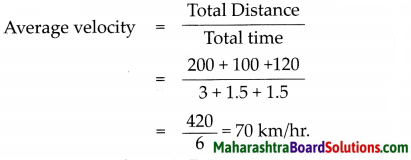Question 2.
See the diagram and calculate the Distance and Displacement travelled by the body from A to I.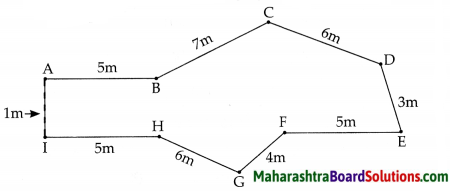Distance travelled =
A → B → C → D → E → F → G → H + I
= 5 + 7 + 6 + 3 + 5 + 4 + 6 + 5
= 41 m
Displacement = A → I in a straight line shortest distance
= 1m